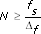# Acquiring a Discrete Time Series (Advanced Signal Processing Toolkit)

LabVIEW 2014 Advanced Signal Processing Toolkit Help

Edition Date: June 2014

Part Number: 372656C-01

»View Product InfoDownload Help (Windows Only)

Signals from physical systems are typically continuous. These real-world signals, such as earthquake waveforms in earthquake monitoring, vibration signals from mechanical devices, or electroencephalogram (EEG) signals, are sampled to form discrete-time representations to enable computer processing. You can sample a continuous time series to form a corresponding discrete time series using data acquisition hardware, such as NI-DAQ devices.

If you have pre-stored data files, use the TSA Read from Files VI to load 1D or 2D arrays of time series data into LabVIEW. The TSA Read from Files VI supports spreadsheet text files and WAV files.Note  Use the Read From Measurement File Express VI to read data from .lvm files. Use the Read Waveform from File VI to read data if you used the Write Waveforms to File VI to save the data.

The following section describes the factors that influence the quality of a discrete time series.

## Factors that Influence a Discrete Time Series

To ensure that the obtained discrete time series accurately represents the information contained in the original continuous time series, you need to consider the following factors when sampling data from a continuous time series.

• Sampling rate—According to the Nyquist sampling theorem, if you want to retain the information that a continuous signal contains, the sampling rate must be greater than twice the highest frequency component in the original signal to avoid frequency aliasing. The Nyquist frequency is half of the sampling rate of the discrete time series.

If you acquire a signal with an NI Dynamic Signal Acquisition (DSA) device, you get automatic aliasing protection. The signal first passes through fixed analog filters that remove the frequency components beyond the range of the analog-to-digital (A/D) converter; then digital anti-aliasing filters automatically adjust the cutoff frequency to remove any frequency component above the Nyquist frequency.

If you acquire a signal with other NI DAQ hardware, you can avoid aliasing with any of the following methods:
• Increase the sampling rate until the Nyquist frequency exceeds the highest frequency component in the signal.
• Apply an external lowpass filter with NI SCXI models.
• Use an inherently bandlimited sensor.
• Number of samples—The number of samples, or the length of a time series, limits how fine the frequency resolution can be. A time series with a large number of samples can provide fine frequency resolution. You usually specify a necessary number of samples based on the following formula:where N is the number of samples fs is the sampling rate Δf required minimum frequency resolution
• Amplitude resolution—The amplitude of a discrete time series for computer processing also is discrete because you usually acquire the time series with an A/D converter. Quantization error of the A/D converter is a correlated and nonlinear impairment that reduces measurement quality. You must make certain that the data has sufficiently fine amplitude resolution.

Not Helpful